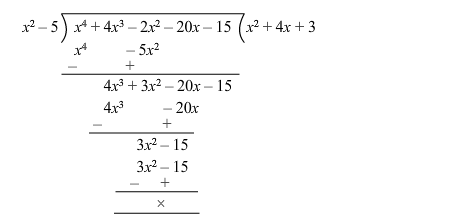# Obtain all other zeros ofQuestion:

Obtain all other zeros of $\left(x^{4}+4 x^{3}-2 x^{2}-20 x-15\right)$ if two of its zeros are $\sqrt{5}$ and $-\sqrt{5}$.

Solution:

The given polynomial is $f(x)=x^{4}+4 x^{3}-2 x^{2}-20 x-15$.

Since $(x-\sqrt{5})$ and $(x+\sqrt{5})$ are the zeroes of $f(x)$, it follows that each one of $(x-\sqrt{5})$ and $(x+\sqrt{5})$ is a factor of $f(x)$.

Consequently, $(x-\sqrt{5})(x+\sqrt{5})=\left(x^{2}-5\right)$ is a factor of $f(x)$.

On dividing $f(x)$ by $\left(x^{2}-5\right)$, we get:$\therefore f(x)=0$

$=>x^{4}+4 x^{3}-7 x^{2}-20 x-15=0$

$=>\left(x^{2}-5\right)\left(x^{2}+4 x+3\right)=0$

$=>(x-\sqrt{5})(x+\sqrt{5})(x+1)(x+3)=0$

$=>x=\sqrt{5}$ or $x=-\sqrt{5}$ or $x=-1$ or $x=-3$

Hence, all the zeros are $\sqrt{5},-\sqrt{5},-1$ and $-3$